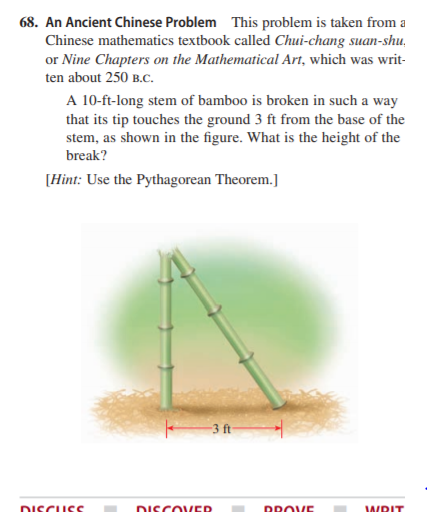# 68. An Ancient Chinese Problem This problem is taken from a Chinese mathematics textbook called Chui-chang suan-shu, or Nine Chapters on the Mathematical Art, which was writ- ten about 250 B.C. A 10-ft-long stem of bamboo is broken in such a way that its tip touches the ground 3 ft from the base of the stem, as shown in the figure. What is the height of the break? [Hint: Use the Pythagorean Theorem.] 3 ft DISCUSS DISCOVER DROVE WRIT

Question

An Ancient Chinese Problem This problem is taken from a
Chinese mathematics textbook called Chui-chang suan-shu,
or Nine Chapters on the Mathematical Art, which was written about 250 b.c.
A 10-ft-long stem of bamboo is broken in such a way
that its tip touches the ground 3 ft from the base of the
stem, as shown in the figure. What is the height of the
break?
[Hint: Use the Pythagorean Theorem.]help_outlineImage Transcriptionclose68. An Ancient Chinese Problem This problem is taken from a Chinese mathematics textbook called Chui-chang suan-shu, or Nine Chapters on the Mathematical Art, which was writ- ten about 250 B.C. A 10-ft-long stem of bamboo is broken in such a way that its tip touches the ground 3 ft from the base of the stem, as shown in the figure. What is the height of the break? [Hint: Use the Pythagorean Theorem.] 3 ft DISCUSS DISCOVER DROVE WRIT fullscreen

### Want to see this answer and more?

Experts are waiting 24/7 to provide step-by-step solutions in as fast as 30 minutes!*

*Response times may vary by subject and question complexity. Median response time is 34 minutes for paid subscribers and may be longer for promotional offers.
Tagged in
Math
Algebra

### Other